# Modal value & Expected valueLet us look at the difference between modal value and expected value. We shall start by saying they are different, albeit close.

Modal value refers to the mode, that is, the value that has the highest probability (chance) of occurring.

Expected value refers to the value, we expect to have, on average.

Before we start, I’ll do a fast recap on Binomial Distribution,by flashing the formulae that we can find on MF26.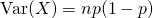The expected value is simply given by.

Now to find the modal value, we have to go through a slightly nasty and long working. You may click and find out.

We have that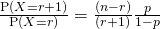. This is what we call the recurrence formula. We consider this to give us the ratio between successive probabilities. And to illustrate how this works, nothing beats an example question.

Consider candies are packed in packets of 20. On average the proportion of candies that are blue-colored is. It is know that the most common number of blue-colored candies in a packet is 6. Use this information to find exactly the range of values thatcan take.

First, most common number is the same as saying the modal/ highest frequency.

This means that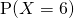is the highest/ largest probability… Let us turn our attention to the recurrence formula now. Ifis the largest, then it means thatand also.

Lets start by looking at the first one…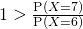But hold on! This looks like the recurrence formula. (ok, in exams, its either you use the recurrence formula or derive on the spot. Both works!)

Now I’ll advice you try the second one (before clicking on answer) on your own, that is,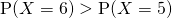.

Now, if the question simply says that the expected number of blue-colored candies in a packet of 20 is 6. Then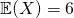We observe that this value actually falls in the range ofwe found.

Not readable? Change text.